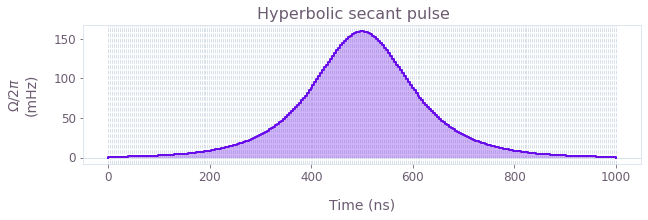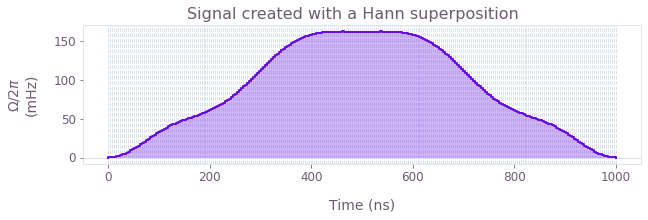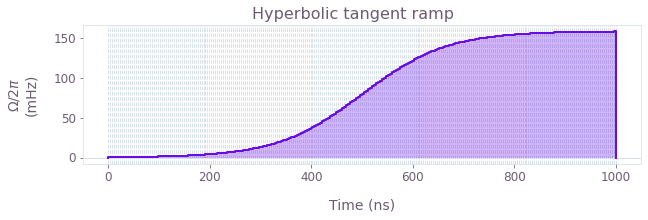•Boulder Opal
• Topics
• Libraries of signals

# Libraries of signals for Boulder Opal

Create parameterized signals for simulation and optimization

The controls used in quantum devices often take the form of signals that are variations over predefined shapes. For example, you can choose to embed your signal in a Gaussian envelope, to make sure that your controls evolve gradually from zero to their peak, and then back to zero again. Boulder Opal equips you with a library of such predefined signal functions, to make it easier for you to create signals according to certain standardized shapes. With them, you only need to provide the minimum required number of parameters that characterize their shapes to create your signals.

The libraries of signals are part of the Boulder Opal Toolkits, which are collections of functions, nodes, and classes that enable you to use Boulder Opal functionality faster and with less code. The signals provided by the Toolkits come in two kinds. The first kind are signals that belong in graphs and can therefore be used in optimization and simulation. The second are signals that are independent of the graph framework and that are suitable to be used with closed-loop optimization and reinforcement learning, because they can easily be exported to external quantum computing backends such as Qiskit, Pyquil, and QUA.

This topic will explain how you can use these two kinds of signals, and show examples of signals that can be generated with them.

The Boulder Opal Toolkits are currently in beta phase of development. Breaking changes may be introduced.

## Graph signals

Signals that are represented as graph nodes can be used as normal controls in a system that you model using the graph representation. You can use these nodes when you want to perform an optimization using qctrl.functions.calculate_optimization or qctrl.functions.calculate_stochastic_optimization, or a simulation using qctrl.functions.calculate_graph.

You can find these signals in the graph.signals namespace of the Graph object in two types of forms: piecewise constant (PWC) signals, which are discretized into segments of constant value, and sampleable tensor-valued function (STF) signals, which are smooth in their shape. These two object types are described and compared in the topic Working with time-dependent functions in Boulder Opal. Most of the signals are available in both PWC and STF output formats, except for those signals that contain discontinuities, which are only available in PWC format. The functions for the two types of signals are distinguished by their suffixes, which are either _pwc or _stf. For most applications, a signal in PWC format is sufficient.

The signals in both formats are usually quite similar, with the inevitable difference that PWC signals are discretized into segments of constant value. For this reason, PWC signals require the parameters segment_count and duration, which are not present in STF signals. The higher the number of segments that you provide in this parameter, the more finely grained the signal will be discretized, and the closer it will be to its STF equivalent.

Some PWC signals contain parts that are constant, such as the flat part of a flat-top pulse. In that case, the relevant PWC signal node, for instance, graph.signals.gaussian_pulse_pwc, accepts a segmentation parameter. With this, you can choose between a uniform segmentation, where the PWC segments are uniformly distributed along the signal's duration, or a minimal segmentation, where the constant parts of the PWC function are represented with a minimal number of segments, reserving most of the segments for the non-constant parts. A uniform segmentation is preferred when combining different signals, while a minimal segmentation leads to a more efficient sampling of the non-constant parts.

Note that many of the parameters that you can pass to the graph signal functions can be Tensors, which means that they can be optimized. For example, if you want to find out what is the width and amplitude of a Gaussian pulse to best perform a certain operation, you can pass these two parameters as optimizable tensors to the graph.signals.gaussian_pulse_pwc function and then run an optimization, using the infidelity of the gate as the cost function. The Boulder Opal optimizer will then tell you which values of the parameters minimize the infidelity.

## Graph-independent signals

Sometimes the kind of optimization that is right for you doesn't involve graphs, as explained in the topic Choosing a control design strategy in Boulder Opal. The library of graph-independent signals contains functions that you can use for this kind of functionality, such as closed-loop optimization and reinforcement learning. For example, suppose that you are interested in optimizing the width or amplitude of a Gaussian pulse used to achieve a certain gate in a real system. In this case, you can use signals from this library together with the Boulder Opal function qctrl.functions.calculate_closed_loop_optimization_step to create different Gaussian-shaped signals and optimize them in your system.

You can access the library of graph-independent signals via the namespace qctrl.signals of the Qctrl object. The functions of this namespace return a Signal object, which contains the duration and shape of the signal. This Signal object can then be discretized and sampled to obtain a series of points that would be appropriate to export to a quantum computing backend with a particular sampling rate or time step.

For example, if you're sending your signals to a quantum computer that requires values sampled every 1 ns, you can use the method export_with_time_step(time_step=1e-9) to obtain an array of values of the signal that meets those requirements. You can then provide these values to the backend to apply this signal to the target quantum device. Similarly, if the quantum computing backend has a specific sampling rate, as opposed to a time step, you can obtain an array of numbers that is adequate to represent the signal for your backend by using the export_with_sampling_rate method of the Signal object.

As you have the liberty to also define the duration of the signal, it is possible that the time step that you provide during discretization might not exactly divide the duration of the signal that you passed. In this case, the total duration of the signal will be rounded up or down to the nearest value that corresponds to a multiple of the time steps. For the other parts of the discretization, the discretized samples correspond to the value of the signal at the middle of the segment that corresponds to the sample.

## Signal types

The libraries of signals have similar kinds of signals shared among them. In the rest of this topic, we present an overview of the main types:

• Pulses: controls that go from zero to a peak, and then back to zero.
• Oscillations: controls that consist of oscillating functions that might have multiple peaks.
• Ramps: controls that monotonically vary from an initial value to a final value.

### Pulses

These are signals that go (either asymptotically or strictly) from zero to a peak, and then back to zero. In the Boulder Opal Toolkits these pulses available are:

The next figure illustrates what a hyperbolic secant pulse created with the Boulder Opal Toolkits looks like.A particular case of these pulses is the square pulse, which has a finite value equal to its amplitude during a specified length of time, and is zero for all the rest of the time. Due to this discontinuity, the square pulse can't be created as an STF. To learn more about square pulses for Boulder Opal, see the reference documentation for graph.signals.square_pulse_pwc and qctrl.signals.square_pulse.

### Oscillations

It is possible to create pulses of many different shapes using superpositions of sines and cosines with different frequencies. In the Boulder Opal Toolkits, these pulses can be found in the form of generic sinusoids or as a Hann series of squared sines with increasingly higher frequencies. To learn more about these oscillatory pulses, see the reference documentation for:

The Hann series in particular can be used as a basis for pulses that are zero at the extremities. The following graph shows an example of a signal created with a Hann superposition.### Ramps

Some signals of the signal library are continuously increasing or decreasing, instead of having a peak-like profile. These are called ramps, and you can learn more about them in the reference documentation for:You can also find usage examples of the library of signals for Boulder Opal in the user guide How to create analytical signals for simulation and optimization. For further information about the library of signals, take a look at their reference documentation.